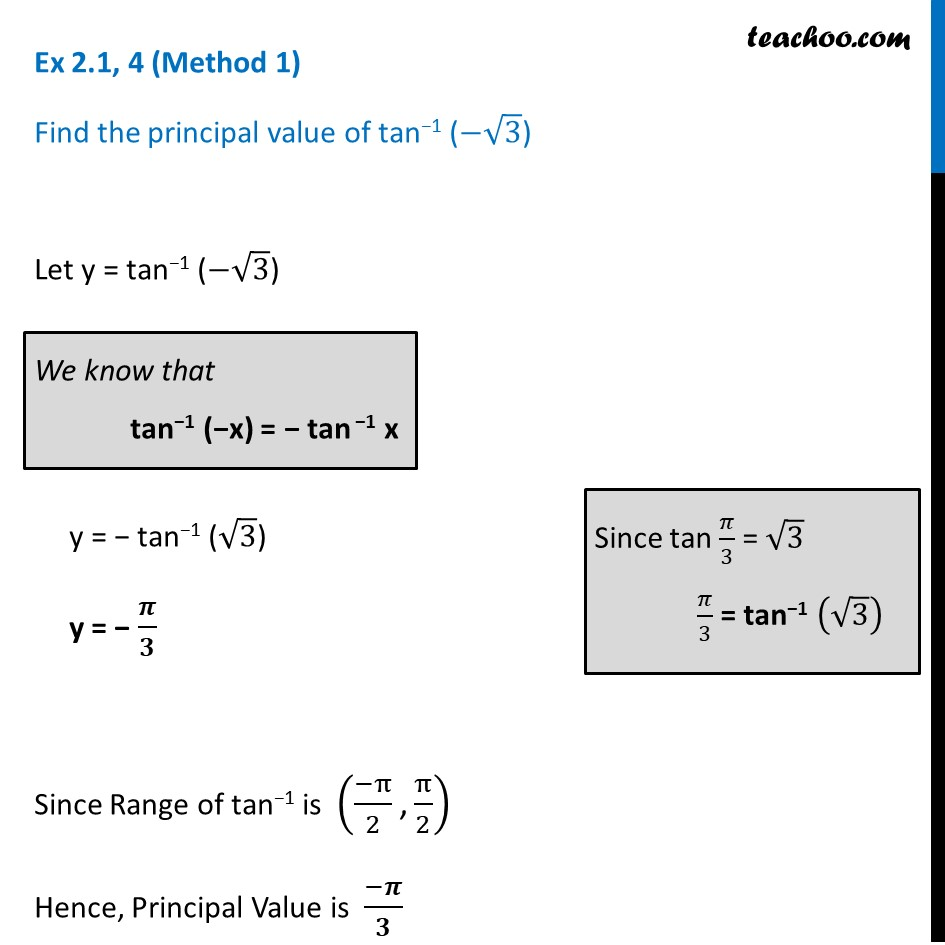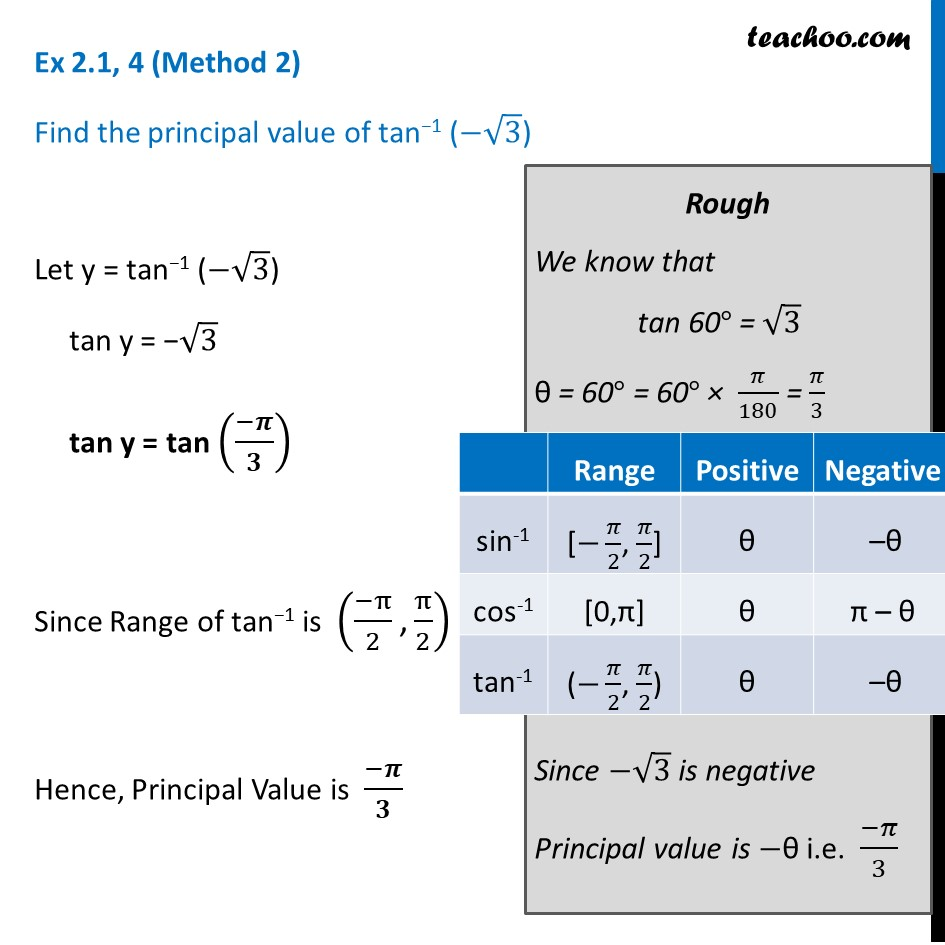Finding principal value

Chapter 2 Class 12 Inverse Trigonometric Functions
Concept wiseLearn in your speed, with individual attention - Teachoo Maths 1-on-1 Class

### Transcript

Ex 2.1, 4 (Method 1) Find the principal value of tan−1 (−√3) Let y = tan−1 (−√3) y = − tan−1 (√3) y = − 𝝅/𝟑 Since Range of tan−1 is ((−π)/2 "," π/2) Hence, Principal Value is (−𝝅)/𝟑 We know that tan−1 (−x) = − tan −1 x Since tan 𝜋/3 = √3 𝜋/3 = tan−1 (√3) Ex 2.1, 4 (Method 2) Find the principal value of tan−1 (−√3) Let y = tan−1 (−√3) tan y = −√3 tan y = tan ((−𝝅)/𝟑) Since Range of tan−1 is ((−π)/2 "," π/2) Hence, Principal Value is (−𝝅)/𝟑 Rough We know that tan 60° = √3 θ = 60° = 60° × 𝜋/180 = 𝜋/3 Since −√3 is negative Principal value is −θ i.e. (−𝜋)/3